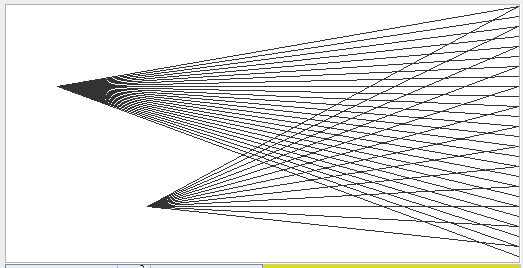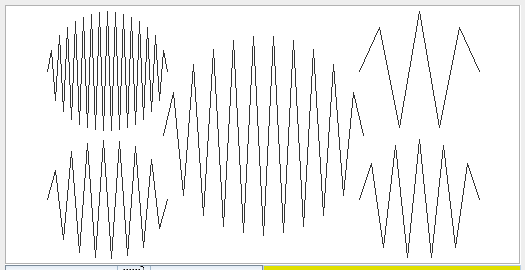# Project 12

In this project we write a program in VM code to draw two nice patterns. Start with the template in pp12.zip.

Your task is to fill in the functions Pattern.lines and Pattern.disk in the file Pattern.vm. The main function Main.main tests all patterns with different parameters. You need to press any key to proceed to the next test. (You can also modify the main function for further testing.)

#### Pattern.lines

The function Pattern.lines(x, y, d) draws lines from the point $$(x,y)$$ to all points $$(511,0), (511,d), (511, 2d), (511, 3d) \ldots$$, as in this screenshot (which shows the result of calling the function two times).#### Pattern.lines

The function Pattern.disk(x, y, r, xstep) fills a disk of radius $$r$$, centered at $$(x,y)$$ with a zig-zag pattern that goes up and down. The $$x$$-coordinate increases by xstep in every zig-step, see the screenshot.You can use all functions of the Jack standard library (see the file "Jack OS API" in projects/09 of the software suite). In particular, you will need to use Math.multiply, Math.sqrt, and Screen.drawLine to draw the lines.

Upload Pattern.vm to our submission server.